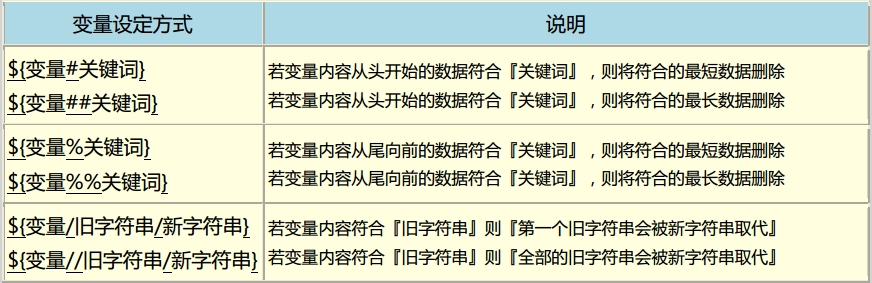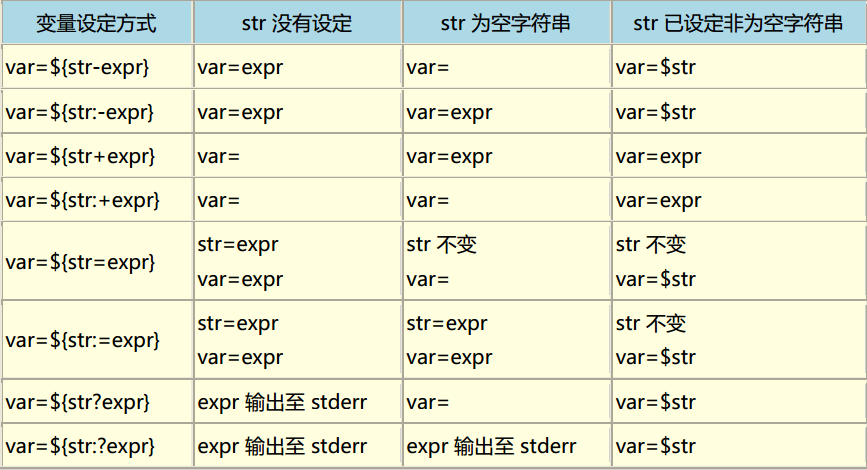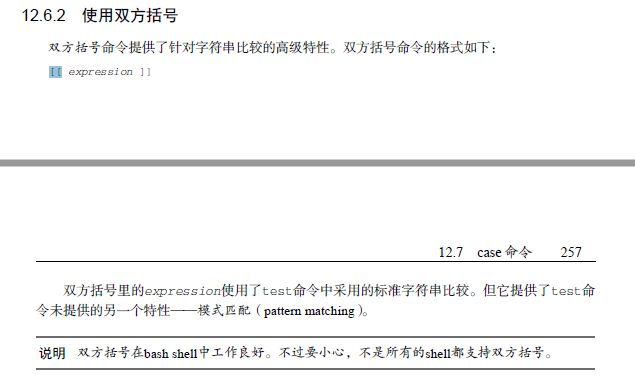# Linux shell 中$()  ，${}，$[]$(())，[ ] (( )) [[ ]]作用与区别

https://blog.csdn.net/x1269778817/article/details/46535729

https://stackoverflow.com/questions/669452/is-double-square-brackets-preferable-over-single-square-brackets-in-ba

https://unix.stackexchange.com/questions/68694/when-is-double-quoting-necessary

$()和   ： 在 bash shell 中，$( ) 与  (反引号) 都是用来做命令替换用(commandsubstitution)的。

${ } ：${ }用于变量替换。一般情况下，$var 与${var} 并没有啥不一样。但是用 ${ } 会比较精确的界定变量名称的范围。比如$ A=B
$echo$AB

$echo${A}B
BB

${ }的功能远不止于此，详见下表。$[] $(()) : 它们是一样的，都是进行数学运算的。支持+ - * / %：分别为 “加、减、乘、除、取模”。但是注意，bash只能作整数运算，对于浮点数是当作字符串处理的。 例：$ a=5; b=7; c=2
$echo$(( a+b*c ))
19
$echo$(( (a+b)/c ))
6
$echo$(( (a*b)%c))
1

$(($a + $b *$c)) 也可得到 19 的结果

[ ] :

1.你必须在左括号的右侧和右括号的左侧各加一个空格，否则会报错。

2.test命令使用标准的数学比较符号来表示字符串的比较，而用文本符号来表示数值的比较。很多人会记反了。使用反了，shell可能得不到正确的结果。

3.大于符号或小于符号必须要转义，否则会被理解成重定向。

(( ))及[[ ]] :posted @ 2018-08-13 00:56  每天一点积累  阅读(15865)  评论(0编辑  收藏  举报